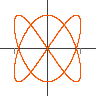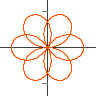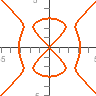Plots Parametric curves like `x=cos(t), y=sin(t)`. Type the equations of the curve into box at the bottom or select one from the list on the right. You can click and drag the mouse to shift the sceen, and use a mouse wheel to zoom, arrow keys also shift and +, -, PageUp, PageDown zoom. Right clicking brings up a menu with other display options.

## Jep plotting appsGraphs
`y=a x^2+b x+c`
JavaScript Version
Java VersionParametric
`x=cos(t), y=sin(t)`
JavaScript Version
Java VersionPolar
`r = cos(3 th/2)`
JavaScript Version
Java VersionComplex
`(z+a)/(z+b)`
JavaScript VersionImplicit
`x^2+y^2=1`
JavaScript Version
Java Version